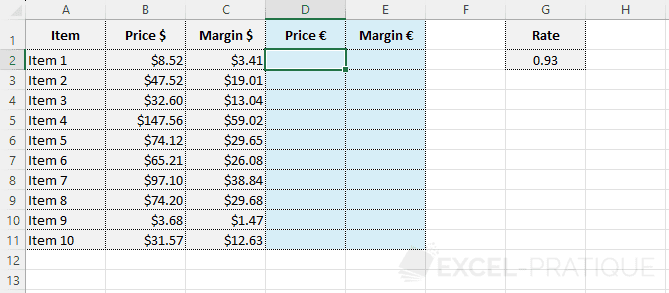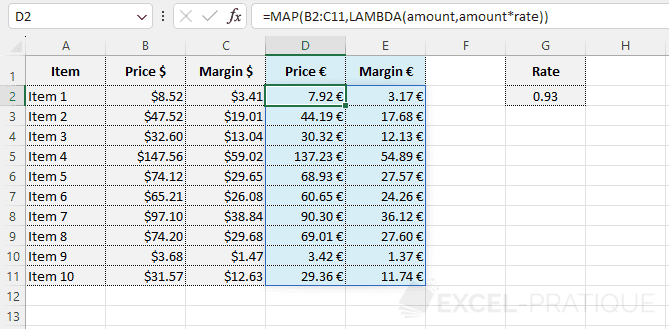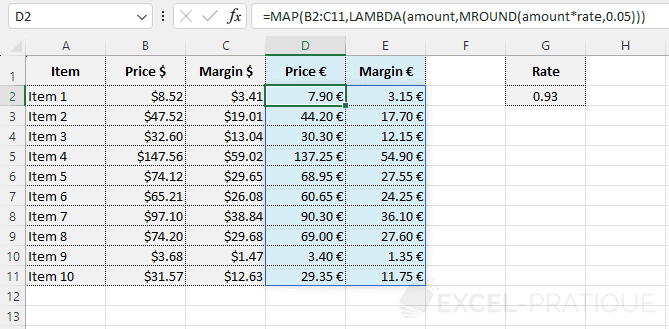# Excel Function: MAP

The MAP function returns an array after applying a LAMBDA function to each value in the array.

Prerequisite: understand the LAMBDA function.

Usage:

`=MAP(array, LAMBDA)`

or

`=MAP(array1, array2, ..., LAMBDA)`

## Example of use

The goal here is to convert the 2 dollar columns into euro with a single formula:Enter the MAP function followed by the array that contains the data to process:

``=MAP(B2:C11``

Then enter the LAMBDA function and choose a variable name (for example "amount"):

``=MAP(B2:C11,LAMBDA(amount``

And finally, enter the formula to apply to each value in the array (knowing that in this example "rate" is a named cell that contains the rate for currency conversion):

``=MAP(B2:C11,LAMBDA(amount,amount*rate))``

The MAP function will then browse the data of the entered array (B2:C11), apply the formula to each amount, and return the new array:If you want to round the calculated amounts to 5 cents, for example, just add the MROUND function to the formula:

``=MAP(B2:C11,LAMBDA(amount,MROUND(amount*rate,0.05)))``